# Forces on Current-Carrying Wires

• Moving charges in magnetic fields experience forces.

• Current in a wire is just a flow of charges.

• If charges are moving perpendicular to magnetic fields, they experience a force which is applied to the wire.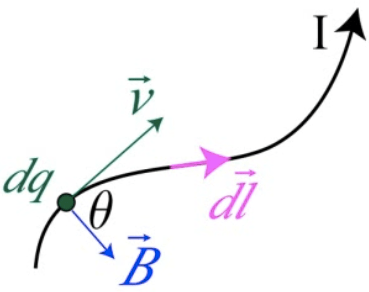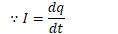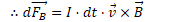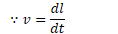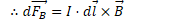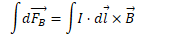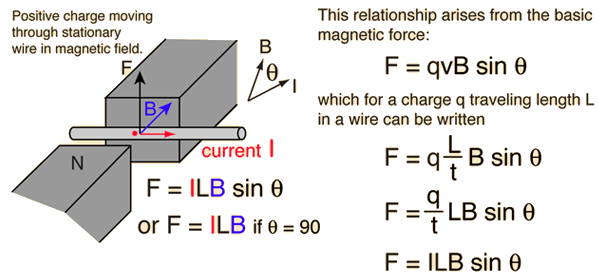# Example 1: Current Through a Wire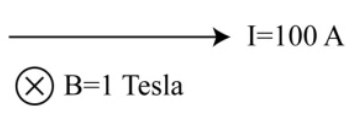• A straight wire of length 1 m carries a current of 100 A through a magnetic field of 1 Tesla. Find the force on the wire

•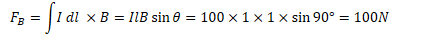# Electric Motors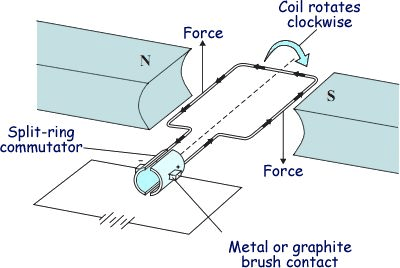# Example 2: Net Torque

• Determine the direction of the net torque on the current-carrying closed circuit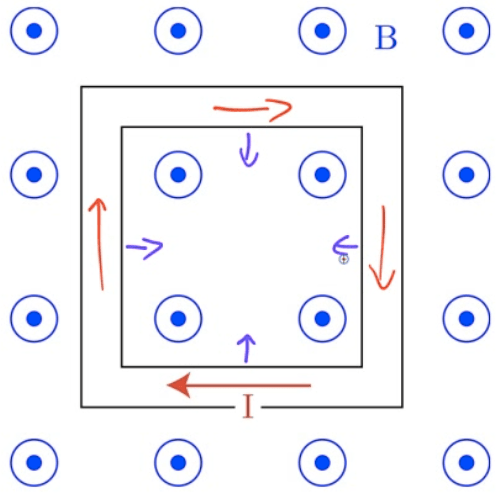# Example 3: Force on a Curved Wire• The curved wire carries constant current I through a uniform magnetic field B as shown. Find the net force acting on the wire.

•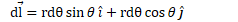•